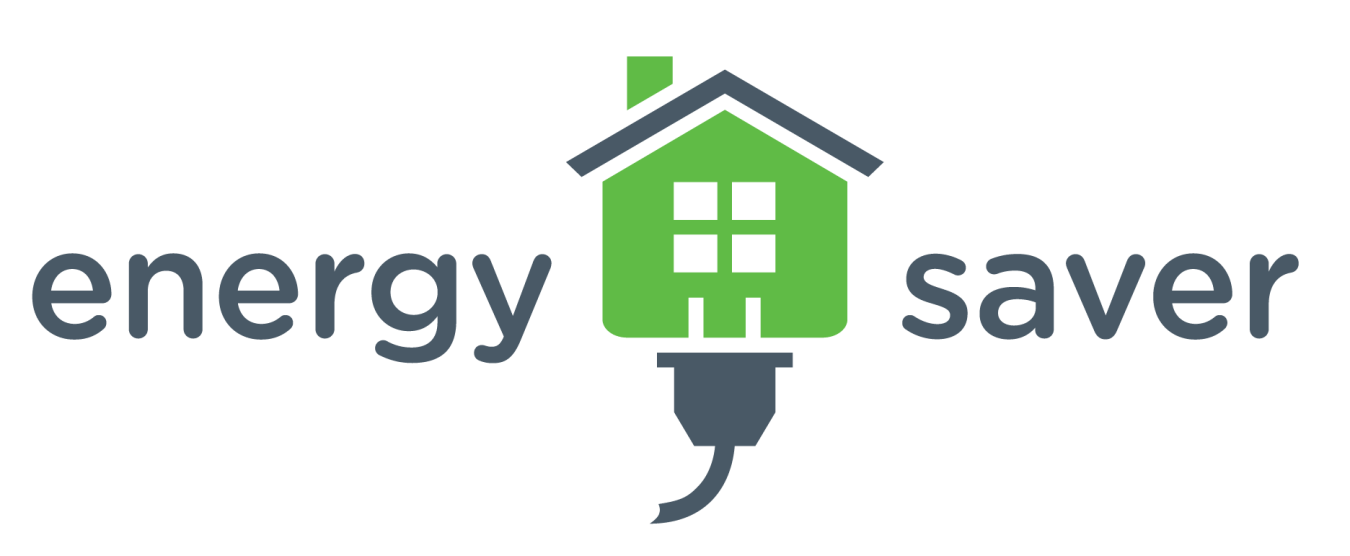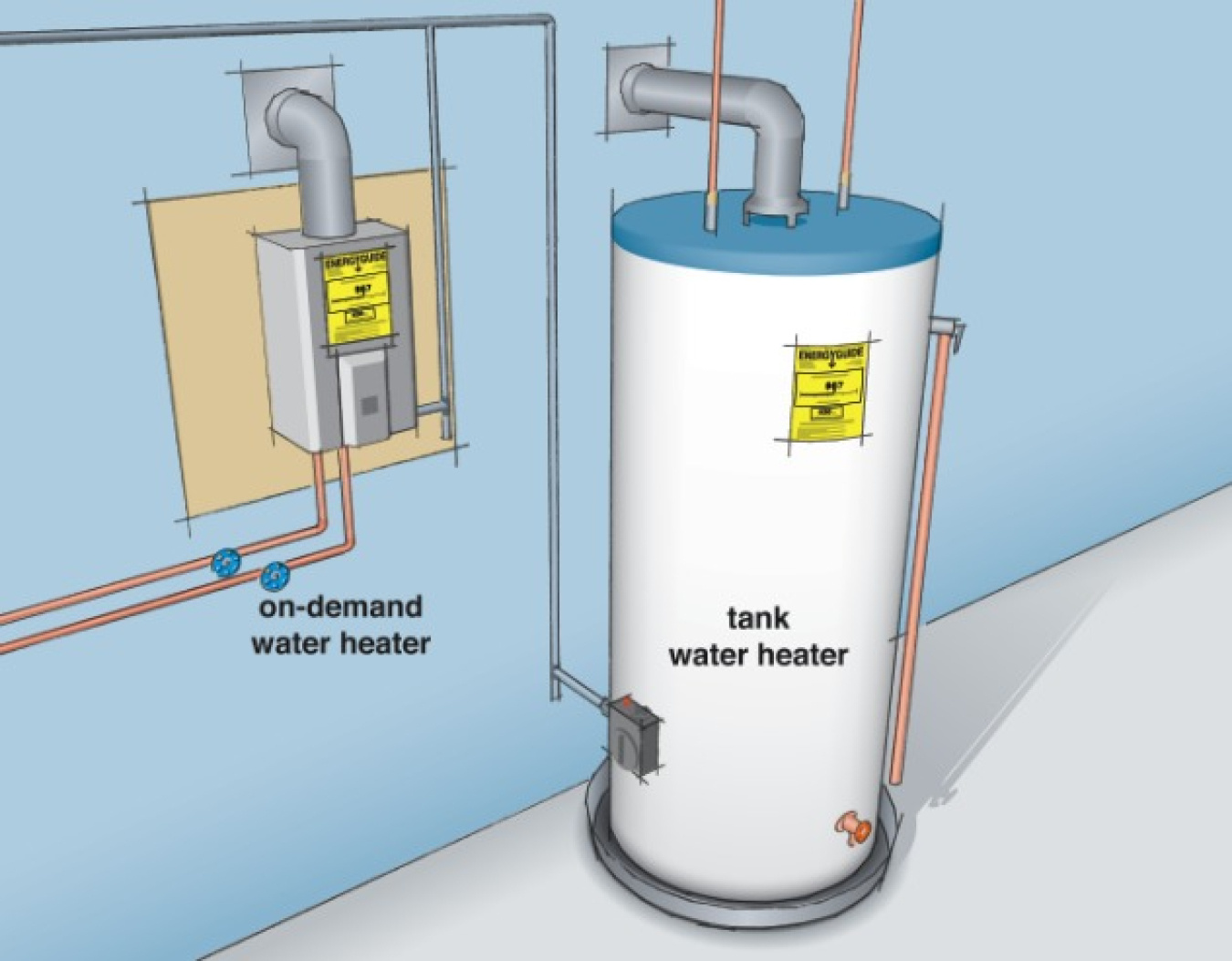When considering a water heater for your home, estimates of its energy efficiency and annual operating cost are shown on the yellow Energy Label. You can then compare costs with other more and/or less energy-efficient models. This will help you determine the dollar savings and payback period of investing in a more energy-efficient model, which will may have a higher purchase price.A water heater's energy efficiency is determined by the energy factor (EF), which is based on the amount of hot water produced per unit of fuel consumed over a typical day. The higher the energy factor, the more efficient the water heater.

## Determining Energy Efficiency of Storage, Demand, and Heat Pump Water Heaters

Use the energy factor to determine the energy efficiency of a storagetankless or demand-type water heater, or heat pump water heater.

The energy factor (EF) indicates a water heater's overall energy efficiency based on the amount of hot water produced per unit of fuel consumed over a typical day. This includes the following:

• Recovery efficiency – how efficiently the heat from the energy source is transferred to the water
• Standby losses – the percentage of heat loss per hour from the stored water compared to the heat content of the water (water heaters with storage tanks)
• Cycling losses – the loss of heat as the water circulates through a water heater tank, and/or inlet and outlet pipes.

The higher the energy factor, the more efficient the water heater. However, higher energy factor values don't always mean lower annual operating costs, especially when you compare fuel sources.

Product literature from a manufacturer usually provides a water heater model's energy factor. Don't choose a water heater model based solely on its energy factor. When selecting a water heater, it's also important to consider size and first hour ratingfuel type, and overall cost.

## Calculating Annual Operating Cost

Before you can choose and compare the costs of various models, you need to determine the correct size water heater for your home. If you haven't done this already, see sizing a new water heater. To estimate the annual operating cost of a storage, demand (tankless or instantaneous), or heat pump water heater, you need to know the following about the model:

• Energy factor (EF) (see above)
• Fuel type and cost (your local utility can provide current rates)

Then, use the following calculations:

#### For gas and oil water heaters

You need to know the unit cost of fuel by Btu (British thermal unit) or therm. (1 therm = 100,000 Btu)

365 days a year X 0.4105 therms/day ÷ EF X Fuel Cost (therm) = estimated annual cost of operation

Example: A natural gas water heater with an EF of .58 and a fuel cost of \$0.00000109/therm

365 X .4105/.58 X \$1.09 (the cost of natural gas on the Energy Label) = \$282

#### For electric water heaters, including heat pump units

You need to know or convert the unit cost of electricity by kilowatt-hour (kWh).

365 days/year x 12.03 kWh/day ÷ EF x Fuel Cost (\$/kWh) = annual cost of operation

Example: A heat pump water heater with an EF of .0 (this is the highest efficiency unit on the market) and an electricity cost of \$0.1301/kWh

365 X 12.03 ÷ 4.0 X \$0.1301 (this is the latest cost of electricity used on the Energy Label) = \$143

The energy usage per day in the above equations is based on the DOE test procedure for hot water heaters, which assumes an incoming water temperature of 58°F, hot water temperature of 135°F, and total hot water production of 64.3 gallons per day, which is the average usage for a household size of three people.

#### Comparing Costs and Determining Payback

Once you know the purchase and annual operating costs of the water heater models you want to compare, you can use the table below to determine the cost savings and payback of the more energy-efficient model(s).

Models Price of Water Heater EF  Estimated Annual Operating Cost
Model A
Model B (higher EF)
Additional cost of more efficient model (Model B)     Price of Model B - Price of Model A = \$Additional Cost of Model B

Estimated annual operating cost savings (Model B)

Model B Annual Operating Cost - Model A Annual Operating Cost = \$Model B's Cost Savings Per Year

Payback period for Model B

\$Additional Cost of Model B/\$Model B's Cost Savings Per Year = Payback period/years

Example:

Comparison of two gas water heaters with a local fuel cost of \$1.09 per therm.

Models Price of Water Heater EF Estimated Annual Operating Cost
Model A \$450 .58 \$282
Model B \$1050 .69 \$237
Additional cost of more efficient model (Model B)     \$1050-\$450=\$600
Estimated annual operating cost savings (Model B)     \$282-\$237=\$45 per year
Payback period for Model B     \$45/\$11 per year = 13.3 years

One thing to consider in the example above is that the high efficiency water heater is a power direct vent unit that can be exhausted using PVC rather than metal ducting. This significantly reduces installation costs and can result in a much shorter payback.

## Other Costs

If you want to include installation and maintenance costs, consult the manufacturer(s) and a qualified contractor to help estimate these costs. These costs will vary among system types and sometimes even from water heater model to model.